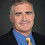## Wednesday, November 06, 2002

### Complexity Class of the Week: SPP, Part II

Previous CCW

Last week we gave the history of the complexity class SPP and described GapP functions. This week we will give a definition of SPP and many of the class' amazing properties.

A language L is in SPP if there is a GapP function f such that

1. If x is in L then f(x)=1.
2. If x is not in L then f(x)=0.
That is if x is in L there is one more accepting than rejecting path. If x is not in L there are the same number of each.

If we used #P functions instead of GapP functions we have the definition of UP. SPP contains UP since every #P function is a GapP function. In fact SPP contains FewP and even Few where we don't believe such languages are in UP.

SPP is the smallest Gap-definable class, i.e., the smallest class that can be defined by GapP functions as above. There are a number of common Gap-definable classes, for example from the Zoo: ⊕P, AWPP, C=P, ModP, ModkP, MP, AmpMP, PP, WPP and of course SPP. SPP is contained in all of these classes. AWPP is the smallest classical class known to contain BQP, the class of problems with efficient quantum algorithms, though it is not known if BQP is itself Gap-definable.

SPP is exactly equal to the low sets for GapP, i.e., SPP is exactly the set of oracles A such that for any NP machine M, the number of accepting minus the number of rejecting paths of M^A(x) is still an (unrelativized) GapP function. This means that SPP is low for all of the Gap-definable classes, for example that ⊕PSPP = ⊕P. This also means that SPP is self-low: SPPSPP = SPP which means SPP is closed under union, complement and in fact any Turing-reduction.

Kobler, Schoning and Toran showed that graph automorphism is in SPP and very recently Arvind and Kurur have show that graph isomorphism is in SPP. This means that graph isomorphism sits in and is in fact low for every Gap-definable class.

The decision tree version of SPP is interesting. A function f on n bits is in this class if there is a polynomial g with polylog degree such that f(x)=g(x) on all x in {0,1}*. All such functions have low deterministic decision tree complexity--the first complexity application of a combinatorial lemma of Nisan and Szegedy. Applications of this result include relativized worlds where SPP does not have complete sets or where P = SPP and the polynomial-time hierarchy is infinite.

1.I think GapP is in FP^#P and so is SPP in P^UP?

1.Yes and no. You can reduce GapP to a #P question but asking whether the gap is one does not reduce to a UP question.

2.Thank you. I was also wondering following. EP is in C=P and the question of C=P is in RP^PromiseSPP which under derandomization would be C=P in P^PromiseSPP? The reasoning is if gap is 0 SPP can detect and if gap is $\neq0$ perhaps there is a gap version of Valiant-Vazirani (unlikely but has it been asked somewhere)?

3.Valiant-Vazirani doesn't seem to work for Gap-P functions. The issues is that a Gap of zero might not stay zero when you eliminate a random set of witnesses.

2.Thank you C=P seems analogous to NP though it is a counting class. But C=P is low for itself and #C=P seems to be #GapP while #NP is different compared to #P. If there was a Vazirani-Valiant perhaps it could be suspicious NP is low for itself and #P=#NP and UP=NP (perhaps there is a barrier to derandomizing because typically if we say derandomizing Valiant Vazirani we think of reduction to a *deterministic* set of instances at least one of which is a single witness instance).

3.Sorry should it be properly x in L iff f(x) is 1 and not in L iff f(x) is 0?